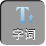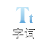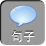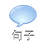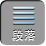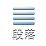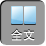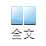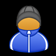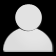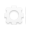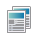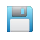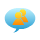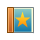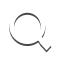-AA+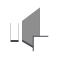1、四边形 ABCD中， AB// CDBECE分别平分∠ ABC、∠ BCD，且点 EAD上，求证： BC= AB+ DC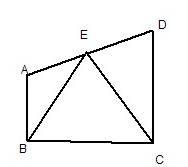BE平分∠ ABC

∴∠ ABE=∠ FBE

∴⊿ ABE≌⊿ FBESAS

∴∠ A=∠ BFE

AB// CD

∴∠ A+∠ D= 180º

∵∠ BFE+∠ CFE= 180º

∴∠ D=∠ CFE

CE= CE

∴⊿ DCE≌⊿ FCEAAS

CD= CF

BC= BF+ CF= AB+ CD

2AB= 4AC= 2DBC的中点， AD是整数，求 AD的长。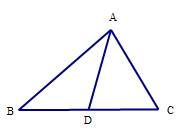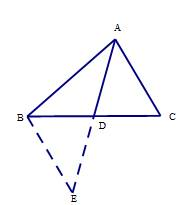DBC的中点（已知）

BD= CB

AD= DE（已知）

ADC=∠ BDE（对顶角）

BD= CB（已证）

∴△ ACD≌△ BEDSAS

AC= BE= 2（全等三角形对应边相等）

AB-BEAEAB+ BE

4-22 AD4+ 2

1AD3

AD是整数

AD= 2

3、如图，在⊿ ABC中， AB= ACDE分别是 ABBC上的点，连结 DE并延长，且与 AC的延长线交于点 F，且 DE= EF，求证： BD= CF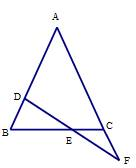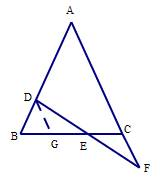DG// AF

∴∠ DGE=∠ ECF

∵∠ GED=∠ CEF

DE= FE

∴⊿ DGE≌⊿ FCE

CF= DG

∴∠ B=∠ ACB

DG// AF

∴∠ DGB=∠ ACB

∴∠ B=∠ DGB

BD= GC

BD= CF

ABC中， AD是∠ CAB的平分线，且 AB= AC+ CD，求证：∠ C= 2B

AB= AC+ CD

AE= AB

AD平分∠ CAB

∴∠ EAD=∠ BAD

AE= AB

EAD=∠ BAD

AD= AD

∴△ ADE≌△ ADB

∴∠ E=∠ B

∴∠ ACD=∠ E+∠ CDE= 2E= 2B